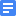Gr3_1-step (60 / 4 = n)Gr3_1-step (563 - 365 = n)Gr3_1-step (679 + 241 = n)Gr3_1-step_4 problems different operationsGr3_2-step ((37 + 35) / 6 = n)Gr3_2-step ((48 + 15) / 7 = n)Gr3_2-step ((120 - 32) / 8 = n)Gr3_2-step ((124 - 88) / 4 = n)Gr3_2-step (4 x 8 + 9 = n)Gr3_2-step (5 x 8 / 4 = n)Gr3_2-step (56 + 39 - 17 = n)Gr3_2-step (120 - 8 x 7 = n)Gr3_2-step (266 + (266 + 144) = n)